Thermodynamics and Propulsion

# 5.6 Muddiest Points on Chapter 5

MP 5..1   Why is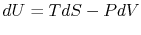always true?

This is a relation between state variables. As such it is not path dependent, only depends on the initial and final states, and thus must hold no matter how we transition from initial state to final state. What is not always true, and what holds only for reversible processes, are the relationsand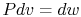. One example of this is the free expansion where, but where the quantitiesand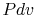(and the integrals of these quantities) are not zero.

MP 5..2   What makes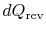different than?

The termdenotes the heat exchange during a reversible process. We use the notationto denote heat exchange during any process, not necessarily reversible. The distinction between the two is important for the reason given above in Section 5.3.

MP 5..3   What happens when all the energy in the universe is uniformly spread, i.e., entropy at a maximum?

I quote from The Refrigerator and the Universe, by Goldstein and Goldstein:

The entropy of the universe is not yet at its maximum possible value and it seems to be increasing all the time. Looking forward to the future, Kelvin and Clausius foresaw a time when the maximum possible entropy would be reached and the universe would be at equilibrium forever afterward; at this point, a state called the heat death'' of the universe, nothing would happen forever after.

The book also gives comments on the inevitability of this fate.

MP 5..4   Why do you rewrite the entropy change in terms of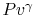?

We have discussed the representation of thermodynamic changes in-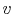coordinates a number of times and it is familiar, as is the idea of the'' process. I want to relate this to the more general expression involving the entropy change Equation (5.5) to show (i) when the simple form applied and (ii) how valid an approximation it was. Using the entropy change, we now have a quantitative metric for doing just that.

MP 5..5   What is the difference between isentropic and adiabatic?

Isentropic means no change in entropy (). An adiabatic process is a process with no heat transfer (). We defined for reversible processes. So generally an adiabatic process is not necessarily isentropic -- only if the process is reversible and adiabatic we can call it isentropic. For example a real compressor can be assumed adiabatic but is operating with losses. Due to the losses the compression is irreversible. Thus the compression is not isentropic.

MP 5..6   In the single reservoir example, why can the entropy decrease?

When we looked at the single reservoir, our system'' was the reservoir itself. The example I did in class had heat leaving the reservoir, so that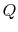was negative. Thus the entropy change of the reservoir is also negative. The second law, however, guarantees that there is a positive change in entropy somewhere else in the surroundings that will be as large, or larger, than this decrease.

MP 5..7   Why does the entropy of a heat reservoir change if the temperature stays the same?

A heat reservoir is an idealization (like an ideal gas, a rigid body, an inviscid fluid, a discrete element mass-spring-damper system). The basic idea is that the heat capacity of the heat reservoir is large enough so that the transfer of heat in whatever problem we address does not appreciably alter the temperature of the reservoir. In grappling with approximations such as this it is useful to think about extreme cases. Therefore, suppose the thermal reservoir is the atmosphere. The mass of the atmosphere is roughlykg (give or take an order of magnitude). Let us calculate the temperature rise due to the heat dumped into the atmosphere by a jet engine during a transcontinental flight. A large gas turbine engine might produce on the order of 100 MW of heat, so that the rise in atmospheric temperature,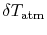, for the heat transferassociated with a 6 hour flight is given bySubstituting for the atmospheric mass and the specific heat gives a value for temperature change of roughly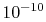K. To a very good approximation, we can say that the temperature of this heat reservoir is constant and we can evaluate the entropy change of the reservoir as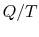.

MP 5..8   How can the heat transfer from or to a heat reservoir be reversible?

We made the assumption that the heat reservoir is very large, and therefore it is a constant temperature heat source or sink. Since the temperature is uniform there is no heat transfer across a finite temperature difference and this heat exchange is reversible. We discussed this in the second example, Heat transfer between two heat reservoirs,'' in Section 5.5.

MP 5..9   How can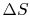be less than zero in any process? Doesn't entropy always increase?

The second law says that the total entropy (system plus surroundings) always increases. (See Section 5.1). This means that either the system or the surroundings can have its entropy decrease if there is heat transfer between the two, although the sum of all entropy changes must be positive. For an isolated system, with no heat transfer to the surroundings, the entropy must always increase.

MP 5..10   If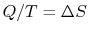for a reservoir, could you addto any size reservoir and still get the same?

Yes, as long as the system you were adding heat to fulfilled the conditions for being a reservoir.

MP 5..11   What is the difference between the isothermal expansion of a piston and the (forbidden) production of work using a single reservoir?

The difference is contained in the word sole in the Kelvin-Planck statement of the second law given in Section 5.1 of the notes.

For the isothermal expansion the changes are:

1. The reservoir loses heat.
2. The system does work(equal in magnitude to).
3. The system changes its volume and pressure.
4. The system changes its entropy (the entropy increases by).

For the forbidden'' process,

1. The reservoir loses heat.
2. The system does work() and that's all the changes that there are. I leave it to you to calculate the total entropy changes (system plus surroundings) that occur in the two processes.

MP 5..12   For the work from a single heat reservoir'' example, how do we know there is no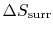?

Our system was the heat reservoir itself. In the example we had heat leaving the reservoir, thuswas negative and the entropy change of the reservoir was also negative. Using the second law, it is guaranteed that somewhere else in the surroundings a positive entropy change will occur that is as large or larger than the decrease of the entropy of the reservoir.

MP 5..13   How does a cycle produce zero? I thought that the whole thing about cycles was an entropy that the designers try to minimize.

The change in entropy during a cycle is zero because we are considering a complete cycle (returning to initial state) and entropy is a function of state (holds for ideal and real cycles!).

The entropy you are referring to is entropy that is generated in the components of a nonideal cycle. For example in a real jet engine we have a non-ideal compressor, a non-ideal combustor and also a non-ideal turbine. All these components operate with some loss and generate entropy -- this is the entropy that the designers try to minimize. Although the change in entropy during a non-ideal cycle is zero, the total entropy change (cycle and heat reservoirs!) is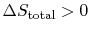. Basically the entropy generated due to irreversibilities in the engine is additional heat rejected to the environment (to the lower heat reservoir). We will discuss this in detail in Section 6.1.

MP 5..14   On the example of free expansion versus isothermal expansion, how do we know that the pressure and volume ratios are the same? We know for each thatand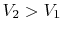.

During the free expansion no work is done and no heat is transferred (insulated system). Thus the internal energy stays constant and so does the temperature. This means that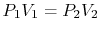holds also for the free expansion and that the pressure and volume ratios are the same when comparing free expansion to reversible isothermal expansion.

MP 5..15   Where did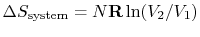come from?

We were using the 1st and 2nd law combined (Gibbs) and in the example discussed there was no change in internal energy (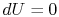). If we then integrateusing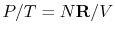(withbeing the number of moles of gas in volumeandbeing the universal gas constant) we obtain.

UnifiedTP